Courses

# Revisial Problems (Past 13 Year) JEE Main (D Block Elements)

## 30 Questions MCQ Test Chemistry for JEE Advanced | Revisial Problems (Past 13 Year) JEE Main (D Block Elements)

Description
This mock test of Revisial Problems (Past 13 Year) JEE Main (D Block Elements) for JEE helps you for every JEE entrance exam. This contains 30 Multiple Choice Questions for JEE Revisial Problems (Past 13 Year) JEE Main (D Block Elements) (mcq) to study with solutions a complete question bank. The solved questions answers in this Revisial Problems (Past 13 Year) JEE Main (D Block Elements) quiz give you a good mix of easy questions and tough questions. JEE students definitely take this Revisial Problems (Past 13 Year) JEE Main (D Block Elements) exercise for a better result in the exam. You can find other Revisial Problems (Past 13 Year) JEE Main (D Block Elements) extra questions, long questions & short questions for JEE on EduRev as well by searching above.
QUESTION: 1

### Only One Option Correct Type Direction (Q. Nos. 1-30) This section contains 30 multiple choice questions. Each question has four choices (a), (b), (c) and (d), out of which ONLY ONE is correct. Q. Which of the following does not relevant with transition elements?

Solution:

A metal is soluble in acid if it displaces H2 from solution, which is determined by the metal’s standard reduction potential. Cu, Ag, Pt and Au cannot displace H2.

QUESTION: 2

### In the following statements about the transition elements are true except that

Solution:

Only Cu shows +1 oxidation state.

QUESTION: 3

### The ground state electronic configuration of the Mn ion in MnO2 is

Solution:

In MnO2, manganese is in Mn4+ form. Hence, the configuration is [Ar] 3d3.

QUESTION: 4

Rutile is an ore of

Solution:

Rutile is a mineral compared primarily of titanium dioxide, TiO2.

QUESTION: 5

Arrange the transition metals Ti, Ni, Mn and La in order of increasing atomic radii,

Solution:

The order of increasing atomic radii is Ni < Mn < Ti < La

QUESTION: 6

For the same transition metal ion, the colour of its compounds will depend upon

Solution:

Colour of the compounds of the same transition metal ion depends upon the nature of ligand attached to it, e.g. [CuCl4]2- is greenish yellow, [Cu(H2O)4]2+ is blue but[Cu(NH3)4]2+ is violet or deep blue.

QUESTION: 7

Isoeiectronic pair of ions is

Solution:

Both have 23 electrons each.

QUESTION: 8

Which oxide is expected to be most acidic?

Solution:

Metal oxide with higher oxidation number exhibit acidic nature.

QUESTION: 9

The enthalpies of hydration of Ca2+ , Mn2+ and Zn2+ follow the order

Solution:

Smaller the ion, more is the hydration and also hydration energy.

QUESTION: 10

Which aqueous solution is coloured?

Solution:

Cobalt +2 ion is blue pink in colour.

QUESTION: 11

Addition of high proportions of manganese makes steel useful in making rails of rail road because manganese

Solution:

Addition of high proportions of Mn gives hardness to steel which is used for making rails of rail roads.

QUESTION: 12

Which of the following set is diamagnetic?

Solution:

Both Hg2+ and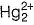do not have unpaired electron.

QUESTION: 13

Which of the following reactions are disproportionation reactions?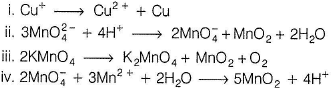Solution:

In (i), (ii) and (iii), same element is undergoing both oxidation and reduction.

QUESTION: 14

Which of the following is amphoteric oxide among Mn2O7, CrO3, Cr2O3, CrO, V2O5, V2O4?

Solution:

Mn2O7 = acidic , CrO3 = acidic , Cr2O3 = amphoteric,
CrO = basic, V2O5 = acidic, V2O4 = amphoteric oxides.

QUESTION: 15

Interstitial compounds are formed when small atoms are trapped inside the crystal lattice of metals. Which of the following is not the characteristic property of interstitial compounds?

Solution:

Interstitial compounds are chemically inert.

QUESTION: 16

Experimentally, it was found that a metal oxide has formula M0.98 O. Metal M present as M2+ and M3+ in its oxide. Fraction of the metal which exists as M3+ would be

Solution:

Consider one mole of the oxide, moles of M = 0.98 and moles of O2- = 1
Let moles of M3+ = x and moles of M2+ = 0.98 - x
During charge balance (0.98 - x) x 2 + 3 x - 2 = 0
On solving, we get x = 0.04,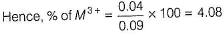QUESTION: 17

In which of the following the hybridisation is different from others?

Solution:

All others have sp3 hybridisation with tetrahedral shape. [Ni(CN)4]2- have dsp2-hybridisation.

QUESTION: 18

Which of the following pairs of transition metal ions are the stronger oxidising agents in aqueous solution?

Solution:

Mn3+ + e- → Mn2+ , E° = + 1.57 V
Co3+ + e- → Co2+ , E° = + 1.97 V

QUESTION: 19

It is possible to change the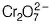ion into the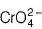ion by the addition of

Solution: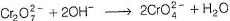QUESTION: 20

The number of moles of dichromate required to oxidise one mole of ferrous and one mole of ferric oxalate respectively are

Solution:

(i) One equivalent dichromate = one equivalent ferrous oxalate or 1/6 mole dichromate = 1/3 mole ferrous oxalate Three moles dichromate = six moles ferrous oxalate = 0.5 mole dichromate
(ii) In ferric oxalate, only oxalate ion undergo oxidation 1/6 mole, dichromate = 1/2 mole ferric oxalate Two mole dichromate = six mole ferrous oxalate or one mole ferrous oxalate = 0.33 mole dichromate

QUESTION: 21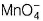oxidises

i. oxalate ion in acidic medium at 333 K and
ii. HCI
For b alanced equations, the ratios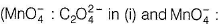HCI in (ii)) respectively are

Solution: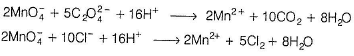QUESTION: 22

Which statement given below is correct regarding dichromate ion ?

Solution: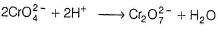QUESTION: 23

Sodium hydrogen sulphite (NaHSO3) is added to a neutral permanganate ion solution. In the reaction that follows, the permanganate ion will be reduced to

Solution:

In neutral or weakly basic medium, perm anganate ion is reduced to MnO2.

QUESTION: 24

KMnO4 acts as an oxidising agent in acidic medium. The number of moles of KMnO4 needed to react with one mole of sulphide ions in acidic solution is

Solution: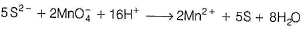QUESTION: 25

Lanthanide for which + 2 and + 3 oxidation states are common is

Solution:

Eu =[Xe] 4f75d0 6s2;Eu shows +2 and + 3 oxidation states.

QUESTION: 26

X + KOH + O2 →Y + H2O

Y + H2SO4 → Z + K2SO4 + MnO2 + H2O . Here X, Y and Z are

Solution:

2MnO2 + 4KOH + O2 → 2K2MnO4 + 2H2O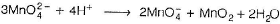QUESTION: 27

Solution:

Promethium is synthetic and radioactive element.

QUESTION: 28

Which of the following ions will exhibit colour in aqueous solutions?

Solution:

Ti3+ have onecf-electron which is responsible for its colour. d-d transition occurs here.

QUESTION: 29

Which one of the following pairs consists of a good oxidising and good reducing agent, respectively?

Solution:

Oxidising agent gains electron and reducing agent loses electrons.
Ce(IV) gain electron to become Ce (III) and hence, strong oxidising agent.
Eu (II) loses one electron to become Eu (III) and hence, act as reducing agent.

QUESTION: 30

The pair of lanthanides with the highest third ionisation energy is

Solution:

Eu = [Xe] 4f75d0 6s2 and Yb = [Xe] 4f145d0 6s2. Removal of 3rd electron requires more energy from these elements.